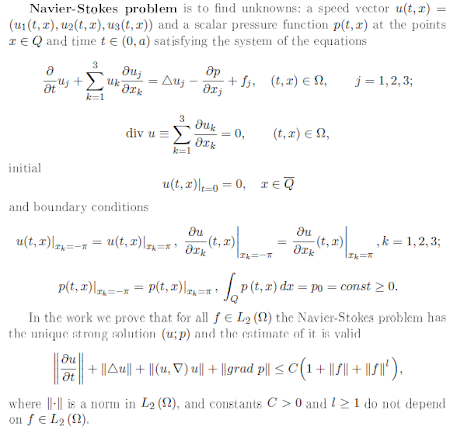### Mathematics problems: Navier-Stokes equations

posted by @ulaulaman about #MillenniumProblems #NavierStokes #MukhtarbayOtelbayev #physics #mathematicsIn fluid mechanics, the Navier-Stokes equations, developed by Claude-Louis Navier and George Gabriel Stokes, describe the motion of a fluid into the space. Given its velocity $\vec{v}$, the pressure $p$, and the kinematic viscosity $\nu$, in presence of an external force $\vec{f}$, the particles' motion in the fluid could be described by the following vector equation: $\frac{\partial \vec{v}}{\partial t} + ( \vec{v} \cdot \vec \nabla ) \vec{v} = -\vec \nabla p + \nu \Delta \vec{v} +\vec{f}(\vec{x},t)$ The trouble is that, to obtain solutions of this equation, we must introduce approximations that simplify the search of them: for example, a major difficulty is to determine the solutions in the presence of some turbulence. To this problem, that it has a physical nature, we must add another mathematical question: the difficulty in proving, given the initial conditions, the existence of smooth solutions for the equations. Given these difficulties, the Clay Mathematics Institute included it in the list of the seven Millennium Problems:
In three space dimensions and time, given an initial velocity field, there exists a vector velocity and a scalar pressure field, which are both smooth and globally defined, that solve the Navier–Stokes equations.Indipendently by the inclusion in the list of Millennium Problems, the research for the solutions of the Navier-Stokes equation has an interesting story that is briefly resumed by Mukhtarbay Otelbayev(1), a mathematician that he seemed to have found the strong solution of the equation. In particular the aspirant solver reports the work of Olga Aleksandrovna Ladyzhenskaya, a soviet mathematician that in 1958(2) proofed the existence and unicity of long time solutions in bi-dimensional Navier-Stokes equations, introducing the inequality that bears her name; and the work of the french mathematician Roger Temam(4). Another important step for Otelbayev was given by Eberhard Hopf(4), who generalized the result by Jean Leray(5) to dimensions lager than 3. Leray proofed the existence of weak solutions for $t > 0$, where the solution at time zero is square summable(6). The solution, however, has a boundary condition, and, as suggested by the kazakh mathematician, the limit determined by Hopf when the size is not less than three is too small in order to use any solving perturbation method and for Otelbayev this is the real reason for which the quest for a strong solution to the Navier-Stokes equations has become a Millennium Problem.
Following this idea, developed over decades (you must read the bibliography, where are many papers by Otelbayev(1)), he reduces the proof of the ecistence of strong solutions to the proof of a new boundary condition(1):It is clear that, before to be sure that the solution is actually arrived, we must wait for the scrutiny of reviewrs of the Clay Mathematics Institute, a work that is complicated by the fact that the paper, apart from the last page, it is not in english, but in cyrillic.
About Navier-Stokes equations, read also: mathworld.wolfram.com, Localisation and compactness properties of the Navier-Stokes global regularity problem by Terrence Tao
About the news, it could be useful to read the discussion on math.stackexchange.com
(1) Mukhtarbay Otelbaev (2013). "The existence of a strong solution to the Navier-Stokes equations". Mathematical Journal Vol 13, Num 4 (50) (pdf - traduzione in inglese in corso di Mikhail Wolfson
(2) Ladyzhensakya, O. A. (1958). "Global solvability of a boundary value problem for the Navier–Stokes equations in the case of two spatial variables". Proceedings of the Academy of Sciences of the USSR 123 (3): 427–429
(3) Vedi, per esempio, Navier-Stokes equations: Theory and numerical analisys (pdf)
(4) Hopf E. (1950). Über die Anfangswertaufgabe für die hydrodynamischen Grundgleichungen. Erhard Schmidt zu seinem 75. Geburtstag gewidmet, Mathematische Nachrichten, 4 (1-6) 213-231. DOI:
(5) Leray J. (1934). Sur le mouvement d'un liquide visqueux emplissant l'espace, Acta Mathematica, 63 (1) 193-248. DOI: (pdf)
(6) Calderón C.P. (1990). Existence of weak solutions for the Navier-Stokes equations with initial data in $L\sp p$, Transactions of the American Mathematical Society, 318 (1) 179-200. DOI: